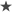Sub Button69_Click()
Dim rng As Range, cell As Range
Set rng = Range("F4:F165")
For Each cell In rng
sMyString = Trim(cell.Value)
If Len(sMyString) > 12 Then
If Right(cell.Value, 3) = "-00" Then cell.Value = Left(cell.Value, Len(cell.Value) - 3) & " rev.00"
'Exit For
End If

Next cell
End Sub